# Test: Probability And Statistics - 7

## 20 Questions MCQ Test Topic-wise Tests & Solved Examples for IIT JAM Mathematics | Test: Probability And Statistics - 7

Description
Attempt Test: Probability And Statistics - 7 | 20 questions in 60 minutes | Mock test for Mathematics preparation | Free important questions MCQ to study Topic-wise Tests & Solved Examples for IIT JAM Mathematics for Mathematics Exam | Download free PDF with solutions
QUESTION: 1

### A car is parked by owner amongst 25 cars in row, not at either end. On his return, he finds that exactly 15 places are still occupied. The probability that both the neighbouring parking places are empty is

Solution: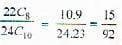QUESTION: 2

### A box contains n enumerated articles. All the articles are taken out one by one at random. The probability that the numbers of the selected articles are in the sequence 1, 2 ........ n is

Solution: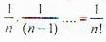QUESTION: 3

### A and B play 12 games of chess of which 6 are won by A, 4 are won by B, and 2 end in a tie. They agree to play a tournament consisting of 3 games. The probability that A and B win alternately is

Solution: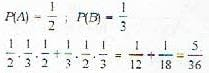QUESTION: 4

In an election, candidate A has 4 chance of winning B has 3 chance, C has, 2 chance and D has, 1 chance. Just before the election C withdraws. The chances of other three candidates are

Solution: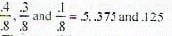QUESTION: 5

An anti-aircraft gun can take a maximum of four shots at a plane moving away from it. The probability of hitting the plane in the first, second, third and fourth shot are 0.4, 0.3, 0.2 and 0.1 respectively. What is the probabilities that the gun hits the plane?

Solution:

The gun hits the plane, its probability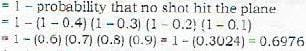QUESTION: 6

If A and B are two independent events in a given sample space and the probability that both A and B occur is 0.16 while the probability that neither occurs is 0.36 the probabilities P[A) and P[B) are respectively

Solution: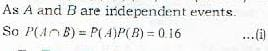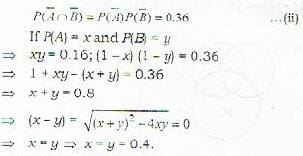QUESTION: 7

A, B and C are independent events such that P(A) = 0.2, P(B) - 0.1 and P(C) = 0.4, then P(A E BE C) equals

Solution: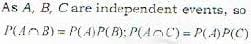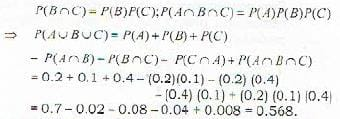QUESTION: 8

Missiles are fired at a target. The probability of each missile hitting the targes is p : the hits are independent of one another. Each missile which hits the target brings it down with probability r. The missiles are fired until the target is brought down or the missile reserve is exhausted. the reserve consists of n missiles (n>2). Defining the event A as at least one missile will remain in reserve", the probability P(A) is:

Solution:

At least one missile will remain in reserve implies that upto (n - 1)th hit target has been brought down.
So, P(A) = 1 - probability that target has not been brought down in the first (n - 1) hits = 1-(1-pr)n-1

QUESTION: 9

For the events A and B to be independent, the probability that both occur is 1/6 and probability that neither of them occur is 1/3. Then the probability of occurrence of A is

Solution:

As A and B are independent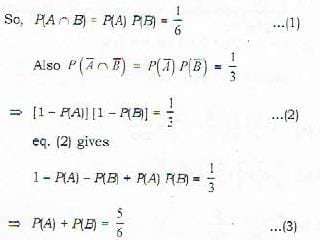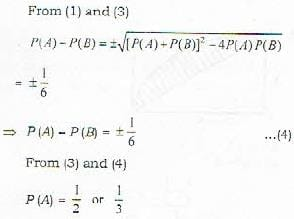QUESTION: 10

For n independent events A1, A2, ...., An, let P(Ai) = 1/(i+1), i = 1, 2 ..., n. Then, the probability that none of the events will occur is

Solution: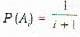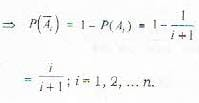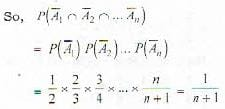QUESTION: 11

Suppose the random variable X has the density function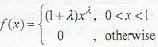The maximum likelihood estimate of λ based on a given random sample X1 = x1,X2 = x2,...,xn = xn is

Solution:

Likelihood function, L is given by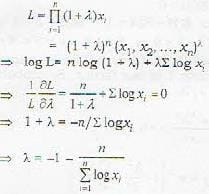QUESTION: 12

Suppose an interactive computer system is proposed for which it is estimated that the mean response time E(T) = 0.5 sec and standard deviation σ = 0.1 sec. Using Chebyshev's inequality, the probability P[|T-0.5| ≥ 0.25] is

Solution:

By chebvshev's inequality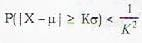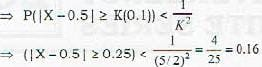QUESTION: 13

X is a normal variate with μ and σ2. The value of E(eX) is

Solution: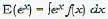...(i)
= Moment generating function of normal variate X with
μ and σ2 is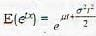By putting t = 1 in equatin (ii)
we get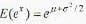QUESTION: 14

A and B throw a die in succession to win a bet with A starting first. Whoever throws '1' first wins an amount of Rs. 110. What are the respective expectations of A and B?

Solution: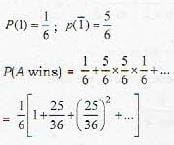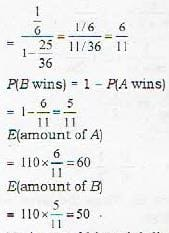QUESTION: 15

For a binomial distribution n = 40 and P = 0.05. The variance for the data is :

Solution:

Variance of binomial distribution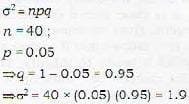QUESTION: 16

For a distribution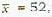variance = 100. The coefficient of variation is:

Solution: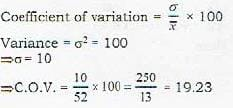QUESTION: 17

For Poisson variate P(x = 1) = P(x = 2). The mean of a x is :

Solution:

If mean is λ1, then for poisson distribution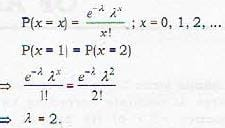QUESTION: 18

The mean of a class is 50 and variance is 9. Then what is the standard error of the marks?

Solution:

Standard error = standard deviation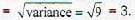QUESTION: 19

If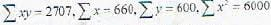then find the coefficient of correlation.

Solution:

Correlation coefficient between random variables x and y is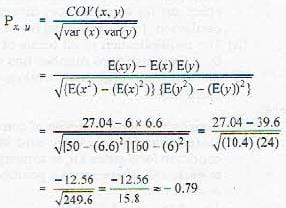QUESTION: 20

If variance is x. If each x term is increased by w, then the new variance is:

Solution:

V(x + c) = v (x); i.e. it is independent of origin.Use Code STAYHOME200 and get INR 200 additional OFF Use Coupon Code